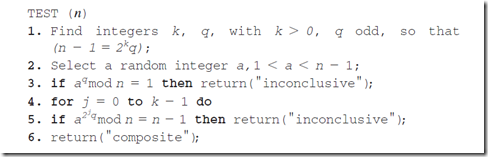## Wednesday, May 9, 2012

### Miller Rabin primality test

I had exercise to implement Miller Rabin primality test

According to my text book, the test algorithms was as followingTest (n)

1. Find integers k,q,d with K>0, q odd, so that (n-1 = 2^k * q);

2. Select a random integer a,1<a<n-1;

3. if a^q mod n = 1 then return probably prime;

4. for j = 0 to k-1 do;

5. if a^2jq mod n = n-1 then return probably prime;

6. return not prime;

I also found another way of the algorithm in http://rosettacode.org/wiki/Miller-Rabin_primality_test as following

`Input: n > 2, an odd integer to be tested for primality;       k, a parameter that determines the accuracy of the testOutput: composite if n is composite, otherwise probably primewrite n − 1 as 2s·d with d odd by factoring powers of 2 from n − 1LOOP: repeat k times:   pick a randomly in the range [2, n − 1]   x ← ad mod n   if x = 1 or x = n − 1 then do next LOOP   for r = 1 .. s − 1      x ← x2 mod n      if x = 1 then return composite      if x = n − 1 then do next LOOP   return compositereturn probably prime`

When I tried both in  C#, both worked as expected

`//textbookpublic static bool IsProbabilyPrime(BigInteger n, int k){    bool result = false;    if (n < 2)        return false;    if (n == 2)        return true;    // return false if n is even -> divisbla by 2    if (n % 2 == 0)        return false;    //writing n-1 as 2^s.d    BigInteger d = n - 1;    BigInteger s = 0;    while (d % 2 == 0)    {        d >>= 1;        s = s + 1;    }    for (int i = 0; i < k; i++)    {        BigInteger a;        do        {            a = RandomIntegerBelow(n - 2);        }        while (a < 2 || a >= n - 2);        if ( BigInteger.ModPow(a,d,n) == 1) return true;        for (int j = 0; j < s - 1; j++)        {            if (BigInteger.ModPow(a,2*j*d,n) == n - 1)                return true;        }        result = false;    }    return result;}//rosettacode.orgpublic static bool IsProbablePrime(this BigInteger source, int certainty){    if (source == 2 || source == 3)        return true;    if (source < 2 || source % 2 == 0)        return false;    BigInteger d = source - 1;    int s = 0;    while (d % 2 == 0)    {        d /= 2;        s += 1;    }    RandomNumberGenerator rng = RandomNumberGenerator.Create();    byte[] bytes = new byte[source.ToByteArray().LongLength];    BigInteger a;    for (int i = 0; i < certainty; i++)    {        do        {            rng.GetBytes(bytes);            a = new BigInteger(bytes);        }        while (a < 2 || a >= source - 2);        BigInteger x = BigInteger.ModPow(a, d, source);        if (x == 1 || x == source - 1)            continue;        for (int r = 1; r < s; r++)        {            x = BigInteger.ModPow(x, 2, source);            if (x == 1)                return false;            if (x == source - 1)                break;        }        if (x != source - 1)            return false;    }    return true;}`